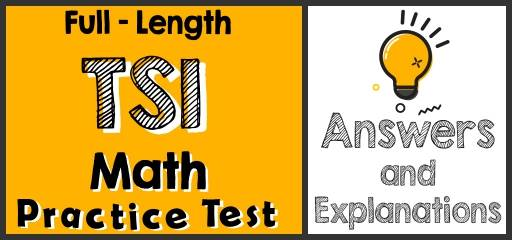# Full-Length TSI Math Practice Test-Answers and ExplanationsDid you take the TSI Math Practice Test? If so, then it’s time to review your results to see where you went wrong and what areas you need to improve.

## TSI Mathematical Reasoning Practice Test Answers and Explanations

1- Choice D is correct
If $$1.05 < x ≤ 3.04$$, then $$x$$ cannot be equal to 3.40. Because: $$3.04<3.40$$

2- Choice B is correct
$$a=8⇒$$ area of triangle is $$=\frac{1}{2} (8×8)=\frac{64}{2}=32$$ cm

3- Choice C is correct
$$66^\circ + 42^\circ = 108^\circ$$
$$180^\circ – 108^\circ = 72^\circ$$
The value of the third angle is $$72^\circ$$.

4- Choice B is correct
Simplify:
$$10 – \frac{2}{3} x ≥ 12 ⇒ – \frac{2}{3} x ≥ 2 ⇒ – x ≥ 3 ⇒ x ≤ – 3$$

5- Choice A is correct
Factor each trinomial $$x^2 – 2x – 8$$ and $$x^2 – 6x + 8$$
$$x^2 – 2x – 8 ⇒ (x – 4)(x + 2)$$
$$x^2 – 6x + 8 ⇒ (x – 2)(x – 4)$$
$$(x – 4)$$ is a factor of both trinomial.

## The Absolute Best Book to Ace the TSI Math Test

6- Choice B is correct
$$\frac{1+b}{6b^2}=\frac{1}{b^2} ⇒(b≠0) b^2+b^3=6b^2⇒b^3-5b^2=0⇒b^2 (b-5)=0⇒b-5=0⇒b=5$$

7- Choice D is correct
Use FOIL (First, Out, In, Last)
$$(x + 7) (x + 5) = x^2 + 5x + 7x + 35 = x^2 + 12x + 35$$

8- Choice A is correct
$$\frac{54}{6}=\frac{27}{3}=9, \frac{48}{6}=\frac{24}{3}=8, \frac{36}{6}=\frac{18}{3}=6, \frac{59}{6}=\frac{59}{6}$$
59 is prime number

9- Choice B is correct
$$x^2 – 64 = 0 ⇒ x^2 = 64 ⇒ x = 8$$

10- Choice C is correct
If $$a = 8$$ then $$b = \frac{8^2}{4} + 4 ⇒ b = \frac{64}{4} + 4 ⇒b = 16 + 4 = 20$$

11- Choice D is correct
tan $$(– π/6) = – \frac{\sqrt{3}}{3}$$

## Best TSI Math Prep Resource for 2022

12- Choice A is correct
$$\frac{\sqrt{32a^5 b^3 }}{\sqrt{2ab^2}}= \frac{4a^2 b\sqrt{2ab}}{b\sqrt{2a}} = 4a^2\sqrt{b}$$

13- Choice D is correct
$$E = 4 + A$$
$$A = S – 3$$

14- Choice C is correct
$$\begin{cases}5x + y = 9\\10x-7y= -18\end{cases} ⇒$$ Multiplication $$(–2)$$ in first equation $$⇒ \begin{cases}-10x- 2y = -18\\10x-7y= -18\end{cases}$$ Add two equations together $$⇒ –9y = –36 ⇒ y = 4$$ then: $$x = 1$$

15- Choice B is correct
$$(x – h)^2 + (y – k)^2 = r^2$$ ⇒ center: (h,k) and radius: r
$$(x – 3)^2 + (y + 6)^2 = 12$$ ⇒ center: $$(3,-6)$$ and radius: $$2\sqrt{3}$$

16- Choice D is correct
c$$(3)=(3)^2+10(3)+30=9+30+30=69$$
$$4×3=12⇒12-69=-57⇒57,000$$ loss

17- Choice B is correct
sinB$$=\frac{the length of the side that is opposite that angle}{the length of the longest side of the triangle}=\frac{4}{5}$$

18- Choice C is correct
$$-7y=-6x-12⇒y=\frac{-6}{-7} x-\frac{12}{-7}⇒y=\frac{6}{7} x+\frac{12}{7}$$

19- Choice D is correct
$$(g – f)(x) = g(x) – f(x) = (– x^2 – 1 – 2x) – (5 + x) – x^2 – 1 – 2x – 5 – x = – x^2 – 3x – 6$$

20- Choice B is correct
$$\frac{|3+x|}{7}≤5⇒|3+x|≤35⇒-35≤3+x≤35⇒-35-3≤x≤35-3⇒-38≤x≤32$$

## More from Effortless Math for TSI Test …

### Are you preparing for the TSI exam and looking for a TSI Math Course?

Try The Ultimate TSI Math Course (+ FREE Worksheets & Tests)! We’re sure this is what you need.

### Need the best TSI math books to improve your math skills?

Top 10 TSI Math Prep Books (Our 2022 Favorite Picks) introduces the Best TSI Math Books on the Market.

## Have any questions about the TSI Test?

### What people say about "Full-Length TSI Math Practice Test-Answers and Explanations - Effortless Math: We Help Students Learn to LOVE Mathematics"?

No one replied yet.

X
52% OFF

Limited time only!

Save Over 52%

SAVE $40 It was$76.99 now it is \$36.99